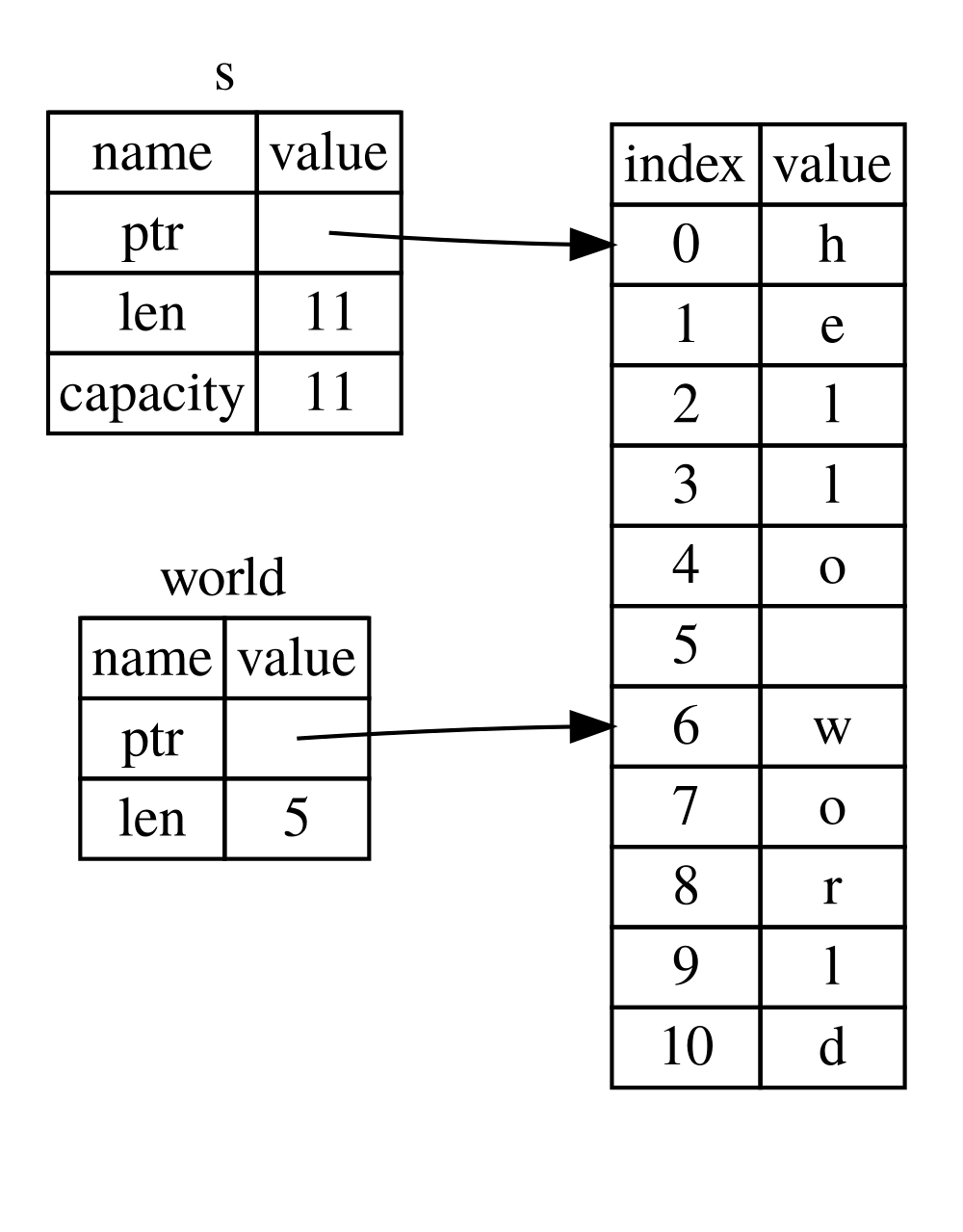# 走进 Rust：切片

Rust 大约 5675 字

## 切片

``fn first_word(s: &String) -> ?``

``````fn first_word(s: &String) -> usize {
let bytes = s.as_bytes();

for (i, &item) in bytes.iter().enumerate() {
if item == b' ' {
return i;
}
}

s.len()
}``````

``let bytes = s.as_bytes();``

``for (i, &item) in bytes.iter().enumerate() {``

`for`循环内，我们使用字节语法来搜索表示空格的字节。如果找到空格，则返回该位置。否则，我们使用`s.len()`返回字符串的长度：

``````    if item == b' ' {
return i;
}
}

s.len()``````

``````fn main() {
let mut s = String::from("hello world");

let word = first_word(&s); // word 变量的值是 5

s.clear(); // 清空字符串，使变量 s 等于 ""

// 这一步，word 变量仍然等于 5，但是没有字符串让我们可以使用这个 5 了。
// word 变量现在等于说是完全无效了。
}``````

``fn second_word(s: &String) -> (usize, usize) {``

## 字符串切片

``````fn main() {
let s = String::from("hello world");

let hello = &s[0..5];
let world = &s[6..11];
}````````````let s = String::from("hello");

let slice = &s[0..2];
let slice = &s[..2];``````

``````let s = String::from("hello");

let len = s.len();

let slice = &s[3..len];
let slice = &s[3..];``````

``````let s = String::from("hello");

let len = s.len();

let slice = &s[0..len];
let slice = &s[..];``````

``````fn first_word(s: &String) -> &str {
let bytes = s.as_bytes();

for (i, &item) in bytes.iter().enumerate() {
if item == b' ' {
return &s[0..i];
}
}

&s[..]
}``````

``fn second_word(s: &String) -> &str {``

``````fn main() {
let mut s = String::from("hello world");

let word = first_word(&s);

s.clear(); // error!

println!("the first word is: {}", word);
}``````

``````\$ cargo run
Compiling ownership v0.1.0 (file:///projects/ownership)
error[E0502]: cannot borrow `s` as mutable because it is also borrowed as immutable
--> src/main.rs:18:5
|
16 |     let word = first_word(&s);
|                           -- immutable borrow occurs here
17 |
18 |     s.clear(); // error!
|     ^^^^^^^^^ mutable borrow occurs here
19 |
20 |     println!("the first word is: {}", word);
|                                       ---- immutable borrow later used here

error: aborting due to previous error

error: could not compile `ownership`.

To learn more, run the command again with --verbose.``````

## 字符串文字是切片

``let s = "Hello, world!";``

`s`的类型是`&str`：它是指向二进制文件的特定点的切片。这也是字符串文字不可变的原因。 `&str`是不可变的引用。

## 字符串切片作为参数

``fn first_word(s: &String) -> &str {``

``fn first_word(s: &str) -> &str {``

``````fn main() {
let my_string = String::from("hello world");

// first_word 传入 `String` s 的切片
let word = first_word(&my_string[..]);

let my_string_literal = "hello world";

// first_word 传入字符串文字的切片
let word = first_word(&my_string_literal[..]);

// 因为字符串文字已经是字符串切片了，所以不需要切片语法了
let word = first_word(my_string_literal);
}``````

## 其他切片

``let a = [1, 2, 3, 4, 5];``

``````let a = [1, 2, 3, 4, 5];

let slice = &a[1..3];``````

## 总结

————        END        ————

Give me a Star, Thanks:)

https://github.com/fendoudebb